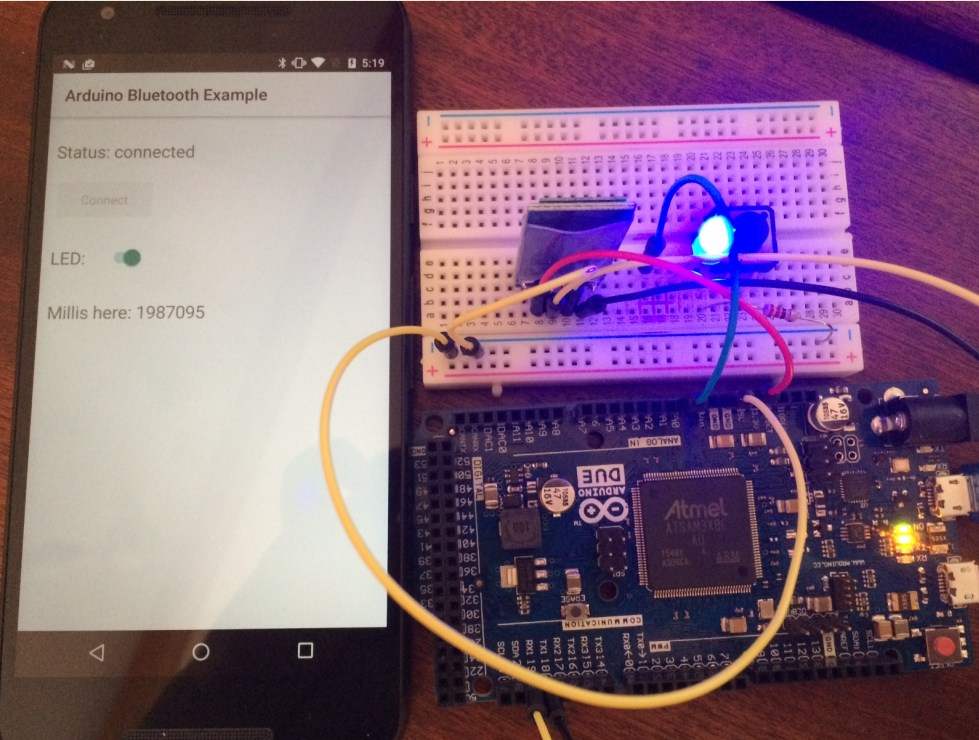# Android Questionshow serial data from arduino

#### wiwit

##### Member
Longtime User
I want to display 5 sensor data from Arduino Uno to Android.
Arduino code:
B4X:
``````void setup() {
Serial.begin(9600);
}

void loop() {

// print out the value you read:
Serial.print("sensor 1 = ");
Serial.println(sensorValue1);
Serial.print("sensor 2 = ");
Serial.println(sensorValue2);
Serial.print("sensor 3 = ");
Serial.println(sensorValue3);
Serial.print("sensor 4 = ");
Serial.println(sensorValue4);
Serial.print("sensor 5 = ");
Serial.println(sensorValue5);
Serial.println(" ");

delay(500);
}``````

Show serial:

sensor 1 = 290
sensor 2 = 320
sensor 3 = 278
sensor 4 = 346
sensor 5 = 347

sensor 1 = 256
sensor 2 = 260
sensor 3 = 290
sensor 4 = 294
sensor 5 = 259

etc...

Android and Arduino connected with BlueTooth HC05

is an example of a simple application to display data coming from Arduino?

#### Erel

##### B4X founder
Staff member
Longtime User
HC 05 Classic Bluetooth